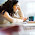## Sunday, June 23, 2013

### An enumerable but not effectively enumerable set

Let's focus on the first half of chapter 5 in Peter Smith's book. First, Smith describes a new characterization of effectively enumerable sets. Second, Smith gives an example of a set of integers that is not effectively enumerable.

Let's define the numerical domain of an algorithm as the set of natural numbers with the following property: when the algorithm takes one of these numbers as input, it terminates and returns a result. Every algorithm has a numerical domain. If the input to some algorithm is not a single natural number, then the numerical domain of this algorithm is the empty set. Otherwise, the numerical domain is the set of those natural numbers on which the algorithm does not crash and does not go into an infinite loop.

It turns out that each numerical domain is effectively enumerable. In fact, the converse is also true, according to the following theorem:
Theorem 1: W is an effectively enumerable set of natural numbers if and only if W is the numerical domain of some algorithm.
Proof sketch of the "only if" direction:
• Let f be the effectively computable function that enumerates W.
• Build the algorithm that, when taking n as input, computes the values f(0), f(1), f(2), ..., f(i) and only stops if and when f(i) = n and then returns, say, 0.
• This algorithm will only stop when its input is in W. Therefore, its numerical domain is W.
Proof sketch of the "if" direction:
• Let A be an algorithm with numerical domain W.
• We know that the set of pairs of natural numbers is effectively enumerable
• So, we build an algorithm A' that takes n as input, computes the nth pair (i,j) and runs algorithm A on input i for exactly j steps.
• If the algorithm A has returned a result after j (or fewer) steps on input i, then A' stops and returns i. Otherwise, A' stops and returns some canonical element of W.
• So, as n increases, all pairs are tested, and algorithm A' enumerates W (repetitions are OK).

So, thanks to Theorem 1, we know that each effectively enumerable set is also the numerical domain of some algorithm.

Since an algorithm is a finite sequence of characters in some formal language, the set of algorithms is effectively enumerable. Therefore, the set of numerical domains is also effectively enumerable. We can thus speak of the ith numerical domain Wi, which is also the ith effectively enumerable set.

Now let's define the set K of natural numbers as follows:

K = { i | i ∈ W}

While K is effectively enumerable (as can be proved in a way similar to the proof sketch of the "if" direction of Theorem 1 above), its complement is not!
Theorem 2: K is not effectively enumerable.
Proof sketch using Cantor's diagonalization method:
• By definition, K = { i | i ∉ W}.
• If 0 ∈ W0 then 0 ∈ K, thus 0 ∉ K. Conversely, if 0 ∉ W0 then 0 ∉ K, thus 0 ∈ K. In all cases, K ≠ W0.
• The same reasoning holds for W1, W2, W3, etc.
• Therefore K is not equal to any of the effectively enumerable sets.

So, K is our first example of a set that is enumerable (it must be, since every subset of ℕ is enumerable) but not effectively so.

In conclusion, the set of "effectively enumerable sets" is exactly the same as the set of "numerical domains of algorithms" (by theorem 1) and we have exhibited one set that is enumerable but is not effectively enumerable.

1.2.